# CO SWBAT use the fundamental identities to simplify

• Slides: 8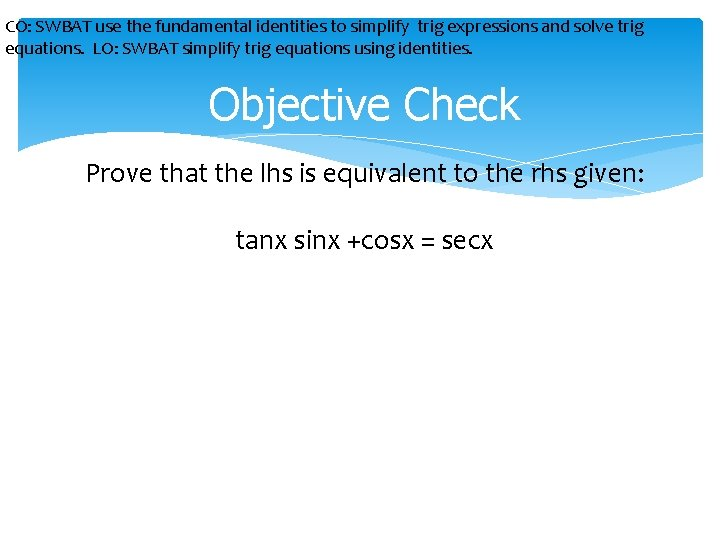CO: SWBAT use the fundamental identities to simplify trig expressions and solve trig equations. LO: SWBAT simplify trig equations using identities. Objective Check Prove that the lhs is equivalent to the rhs given: tanx sinx +cosx = secx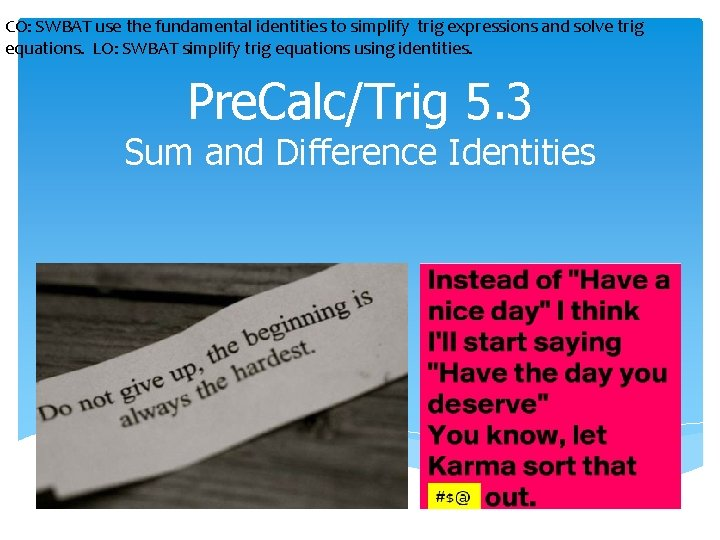CO: SWBAT use the fundamental identities to simplify trig expressions and solve trig equations. LO: SWBAT simplify trig equations using identities. Pre. Calc/Trig 5. 3 Sum and Difference Identities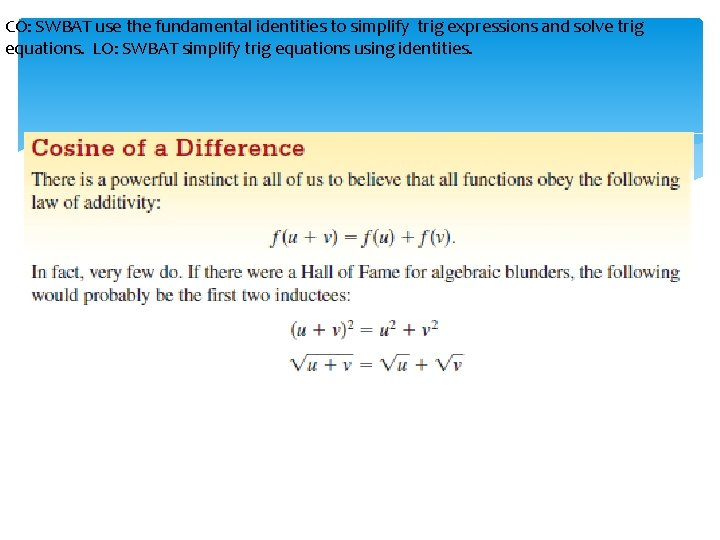CO: SWBAT use the fundamental identities to simplify trig expressions and solve trig equations. LO: SWBAT simplify trig equations using identities.CO: SWBAT use the fundamental identities to simplify trig expressions and solve trig equations. LO: SWBAT simplify trig equations using identities.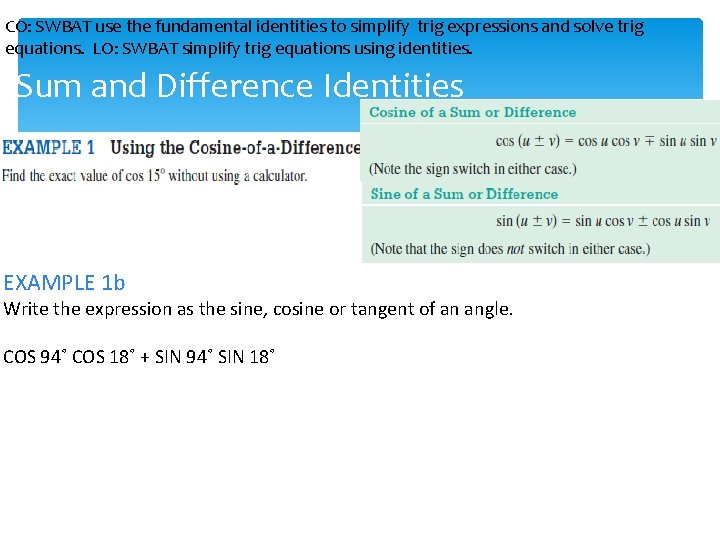CO: SWBAT use the fundamental identities to simplify trig expressions and solve trig equations. LO: SWBAT simplify trig equations using identities. Sum and Difference Identities EXAMPLE 1 b Write the expression as the sine, cosine or tangent of an angle. COS 94˚ COS 18˚ + SIN 94˚ SIN 18˚CO: SWBAT use the fundamental identities to simplify trig expressions and solve trig equations. LO: SWBAT simplify trig equations using identities. Sum and Difference IdentitiesCO: SWBAT use the fundamental identities to simplify trig expressions and solve trig equations. LO: SWBAT simplify trig equations using identities. Sum and Difference Identities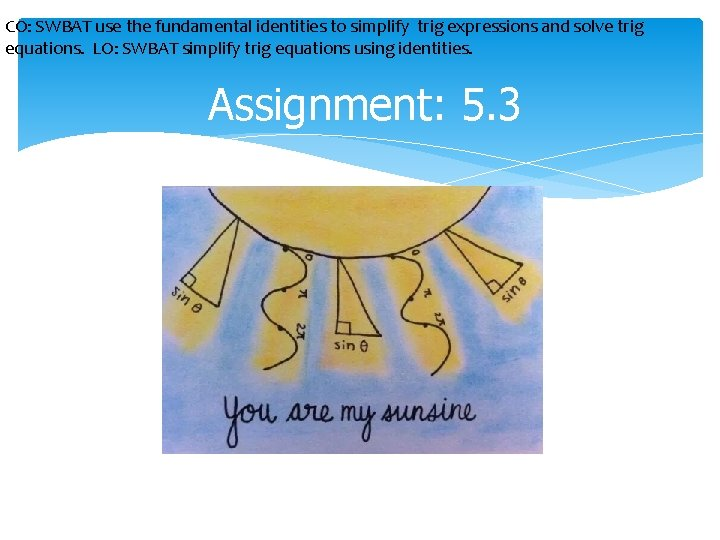CO: SWBAT use the fundamental identities to simplify trig expressions and solve trig equations. LO: SWBAT simplify trig equations using identities. Assignment: 5. 3